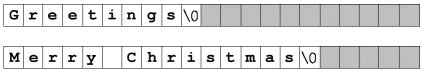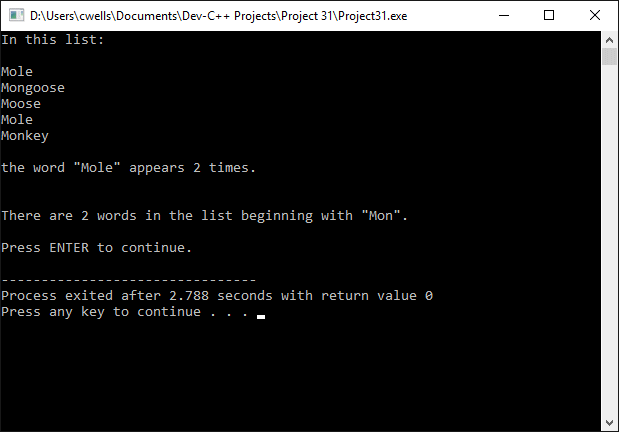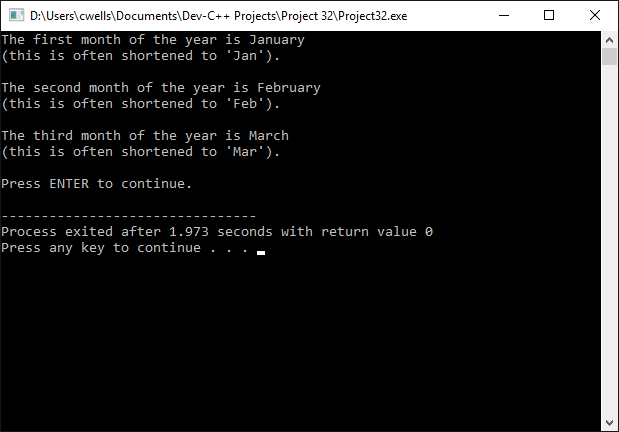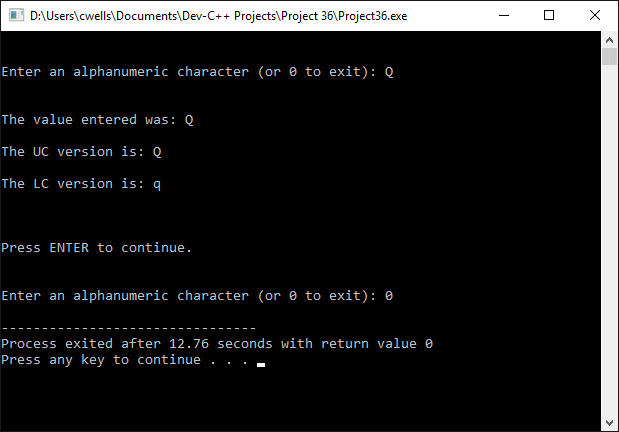Strings and Characters

Before investigating the implementation of strings in C++, it is probably a good idea to familiarse yourself with encoding schemes. Because strings are essentially arrays of characters, it is important to realise that each of the commonly used encoding schemes uses a specific character type.

The oldest type of character encoding scheme uses a single byte to represent each character. The ASCII encoding scheme falls into this category. Strings that are encoded in this way consist of an array of single-byte characters, followed by the null character (a byte with the value of 0), which marks the end of the string.

Another character scheme employs a multi-byte character set to represent characters in which each character is one or more bytes long. In Windows systems, the largest multi-byte character uses two bytes. As with single byte character encoding schemes, a single null byte marks the end of a string.

The last encoding scheme discussed here is Unicode, an encoding standard in which all characters are two bytes long. A Unicode string is terminated by two zero bytes (the encoding of the value 0).

The char data type is used to represent single byte characters, and to manipulate double byte characters (more about this later). Unicode characters are represented by the wchar_t type, and Unicode characters and string literals are prefixed with L, as shown below:

wchar_t myChar = L'1';     // 2 bytes (0x0031)
wchar_t* myString = L"Hello";  // 12 bytes

Although the C++ standard library implements a powerful string class that can be used to manipulate strings of characters, we also often represent strings as arrays of char elements. For example, the following character array variable can be used to hold a string up to twenty characters in length:

char myString

The string held in the character array myString may have up to twenty-one characters including the terminating null character ('\0'). The two character arrays shown below, for example, both have space for twenty-one characters, but the strings they represent have different lengths.Character arrays

The null character (written as a backslash followed by a zero) indicates to the compiler that it has reached the end of the string. The character elements that follow the null character in the character array are ignored. A character array, like any other variable, can be initialised with a series of array elements, as shown in the following program statement:

char myString = {'H', 'e', 'l', 'l', 'o', '\0'};

Strings that consist of character arrays can also be initialised using string literals, however, as shown below:

char myString = "Hello";

Strings of characters enclosed between double quotation marks (") are known as string literals. String literals have a null character appended automatically after the last character. A string variable intended to hold a string literal of up to n characters, therefore, should be declared as having n+1 elements to allow for the final null terminating character. Note, however, that a string literal may not be assigned to a character array using the assignment operator after it has been declared.

String handling functions

Standard C/C++ string handling functions like strcpy() can only be used with single-byte strings, although there are alternative versions of these functions, such as wcscpy(), for use with Unicode strings. Declarations for most of these string handling functions are to be found in the string.h header file. Some of them are described in the following table.

String handling functions in <string.h>
FunctionDeclarationDescription
strcat()char* strcat(char* destination, const char* source)Adds string source to the end of destination.
strncat()char* strncat(char* destination, const char* source, size_t n)Appends the first n characters of source to destination, and returns a pointer to destination.
strchr()char* strchr(const char* s, int c)Returns a pointer to the first occurrence of c in string s (or NULL if c is not found).
strrchr()char* strrchr(const char*s, int c)Returns a pointer to the last occurrence of c in string s.
strcmp()int strcmp(const char* destination, const char* source)Compares destination and source and returns a number less than, equal to or greater than zero, dependent on whether destination is less than, equal to, or greater than source.
strncmp()int strncmp(const char* destination, const char* source, size_t n)Compares destination with the first n characters of source. Returns a number less than, equal to or greater than zero, dependent on whether destination is less than, equal to, or greater than the specified substring in source.
strcpy()char* strcpy(char* destination, const char* source)Copies source into destination, and returns a pointer to destination.
strncpy()char* strncpy(char* destination, const char*source, size_t n)Copies the first n characters of source into the first n characters of destination.
strlen()size_t strlen(const char* s)Returns the number of characters in string s (excluding the NULL terminating character).
strstr()char* strstr(const char* destination, const char* source)Returns the address of the first occurrence of string source within destination (or NULL if source is not found).

The strcat() and strncat() functions

The following short program demonstrates the use of both the strcat() function and the strcat() function.

// Example Program 1

#include <string.h>
#include <iostream>
using namespace std;

int main()
{
char string1 = "The date and time is: ";
char string2 = "01-Jul-2008 12:46:23";
char string3 = "The date is: ";
string s;

strcat(string1, string2);
cout << string1 << "\n\n";
strncat(string3, string2, 11);
cout << string3 << "\n\n";
cout << "\n\nPress ENTER to continue.";
getline( cin, s );
return 0;
}The output from example program 1

The strchr() and strrchr() functions

The following short program demonstrates the use of both the strchr() function and the strrchr() function.

// Example Program 2

#include <string.h>
#include <iostream>
using namespace std;

int main()
{
char str = "\"The boy stood on the burning deck.\"";
char *charPtr;
int n;
string s;

cout << "There are two occurrences of the letter \"b\" ";
cout << "in the following sentence: \n\n";
cout << str << "\n\n"; charPtr = strchr(str, 'b');
n = charPtr - str;
cout << "The first is at position " << n << ".\n\n";
charPtr = strrchr(str, 'b');
n = charPtr - str;
cout << "The second is at position " << n << ".";
cout << "\n\nPress ENTER to continue.";
getline( cin, s );
return 0;
}The output from example program 2

The strcmp() and strncmp() functions

The following short program demonstrates the use of both the strcmp() function and the strncmp() function.

// Example Program 3

#include <string.h>
#include <iostream>
using namespace std;

int main()
{
char* animal[] = {"Mole", "Mongoose", "Moose", "Mole", "Monkey"};
string s;
int x;

cout << "In this list:\n\n";
x = 0;
for(int i=0; i<5; i++)
{
cout << animal[i] << "\n";
if(strcmp(animal[i], "Mole") == 0) x++;
}
cout << "\n" << "the word \"Mole\" appears ";
cout << x << " times. \n\n";
x = 0;
for(int i=0; i<5; i++)
{
if(strncmp(animal[i], "Mon", 3) == 0) x++;
}
cout << "\n" << "There are " << x;
cout << " words in the list beginning with \"Mon\".";
cout << "\n\nPress ENTER to continue.";
getline( cin, s );
return 0;
}The output from example program 3

The strcpy() and strncpy() functions

The following short program demonstrates the use of both the strcpy() function and the strncpy() function.

// Example Program 4

#include <string.h>
#include <iostream>
using namespace std;

int main()
{
char str01 = "January";
char str02 = "February";
char str03 = "March";
char longMon01, longMon02, longMon03;
char shortMon01 = "", shortMon02 = "", shortMon03 = "";
string s;

strcpy(longMon01, str01);
strcpy(longMon02, str02);
strcpy(longMon03, str03);
strncpy(shortMon01, str01, 3);
strncpy(shortMon02, str02, 3);
strncpy(shortMon03, str03, 3);
cout << "The first month of the year is " << longMon01 << "\n";
cout << "(this is often shortened to '" << shortMon01 << "').\n\n";
cout << "The second month of the year is " << longMon02 << "\n";
cout << "(this is often shortened to '" << shortMon02 << "').\n\n";
cout << "The third month of the year is " << longMon03 << "\n";
cout << "(this is often shortened to '" << shortMon03 << "').";
cout << "\n\nPress ENTER to continue.";
getline( cin, s );
return 0;
}The output from example program 4

The strlen() function

The following short program demonstrates the use of the strlen() function.

// Example Program 5

#include <string.h>
#include <iostream>
using namespace std;

int main()
{
char strCity1 = "London";
char strCity2 = "Paris";
char strCity3 = "Washington";
int len1, len2, len3;
string s;

len1 = strlen(strCity1);
len2 = strlen(strCity2);
len3 = strlen(strCity3);
cout << "The capital of England is: " << strCity1 << "\n";
cout << strCity1 << " has " << len1 << " letters.\n\n";
cout << "The capital of France is: " << strCity2 << "\n";
cout << strCity2 << " has " << len2 << " letters.\n\n";
cout << "The capital of the USA is: " << strCity3 << "\n";
cout << strCity3 << " has " << len3 << " letters.\n\n";
cout << "\n\nPress ENTER to continue.";
getline( cin, s );
return 0;
}The output from example program 5

The strstr() function

The following short program demonstrates the use of the strstr() function.

// Example Program 6

#include <string.h>
#include <iostream>
using namespace std;

int main()
{
char strAirports = "LHR, LGW, MAN, STN, BHX, GLA, EDI, LTN, BFS, BRS";
char airportCode = "";
char *charPtr;
int n;
string s;

cout << "Please enter a UK airport code: ";
cin >> airportCode;
getline( cin, s );
charPtr = strstr(strAirports, airportCode);
if (charPtr == NULL)
{
cout << "\n\nThat code is not one of the top 10 UK airports.";
}
else
{
n = charPtr - strAirports;
n = n/5 + 1;
cout << "\n\nThat code is the number " << n << " UK airport.";
}
cout << "\n\nPress ENTER to continue.";
getline( cin, s );
return 0;
}The output from example program 6

Character handling functions

The character handling functions described in the table below are declared in the ctype.h header file. With the exception of the toupper() and tolower() functions, all the functions return Boolean (true/false) values.

Character functions in <ctype.h>
FunctionDeclarationDescription
isalnum()int isalnum(int c)Returns a non-zero value if c is alphanumeric.
isalpha()int isalpha(int c)Returns a non-zero value if c is alphabetic.
iscntrl()int iscntrl(int c)Returns a non-zero value if c is a control character.
isdigit()int isdigit(int c)Returns a non-zero value if c is a digit (0-9).
isgraph()int isgraph(int c)Returns a non-zero value if c is a graphic character.
islower()int islower(int c)Returns a non-zero value if c is a lower case character (a-z).
isprint()int isprint(int c)Returns a non-zero value if c is a printable character.
ispunct()int ispunct(int c)Returns a non-zero value if c is a punctuation character.
isspace()int isspace(int c)Returns a non-zero value if c is a white space characters or one of the escape sequences: '\f', '\n', '\r', '\t', or '\v'.
isupper()int isupper(int c)Returns a non-zero value if c is an upper-case character (A-Z).
isxdigit()int isxdigit(int c)Returns a non-zero value if c is a hexadecimal character.
tolower()int tolower(int c)Returns the lower case version of c.
toupper()int toupper(int c)Returns the upper case version of c.

The short program below illustrates the use of the character functions.

// Example Program 7

#include <ctype.h>
#include <string.h>
#include <iostream>
using namespace std;

int main()
{
unsigned int charCode;
string s;

while (charCode < 128)
{
cout << "\nEnter a character code (0-127) or >=128 to exit: ";
cin >> charCode;
getline( cin, s );
if (charCode >= 128) return 0;
cout << "\n";
cout << "isalnum : " << isalnum(charCode) << "\n";
cout << "isalpha : " << isalpha(charCode) << "\n";
cout << "iscntrl : " << iscntrl(charCode) << "\n";
cout << "isdigit : " << isdigit(charCode) << "\n";
cout << "isgraph : " << isgraph(charCode) << "\n";
cout << "islower : " << islower(charCode) << "\n";
cout << "isprint : " << isprint(charCode) << "\n";
cout << "ispunct : " << ispunct(charCode) << "\n";
cout << "isspace : " << isspace(charCode) << "\n";
cout << "isupper : " << isupper(charCode) << "\n";
cout << "isxdigit : " << isxdigit(charCode) << "\n";
if (!isprint(charCode)) cout << "The character cannot be printed.";
else if (isspace(charCode)) cout << "The character is a space.";
else (cout << "The character is: \"" << (char)charCode) << "\"";
cout << "\nPress ENTER to continue.";
getline( cin, s );
}
}The output from example program 7

The short program below illustrates the use of the toupper() and tolower() functions. Note that these functions only convert a character to upper or lower case if the character is not already of the required case.

// Example Program 8

#include <ctype.h>
#include <string.h>
#include <iostream>
using namespace std;

int main()
{
string s;
char c;
while (1)
{
cout << "\n\nEnter an alphanumeric character (or 0 to exit): ";
cin >> c;
getline( cin, s );
if (c == '0') return 0;
else
{
cout << "\n\nThe value entered was: " << c << "\n\n";
if(!isalnum(c)) cout << "This value is not alphanumeric.\n\n";
else
{
cout << "The UC version is: " << (char)toupper(c) << "\n\n";
cout << "The LC version is: " << (char)tolower(c) << "\n\n";
}
}
cout << "\n\nPress ENTER to continue.";
getline( cin, s );
}
}The output from example program 8